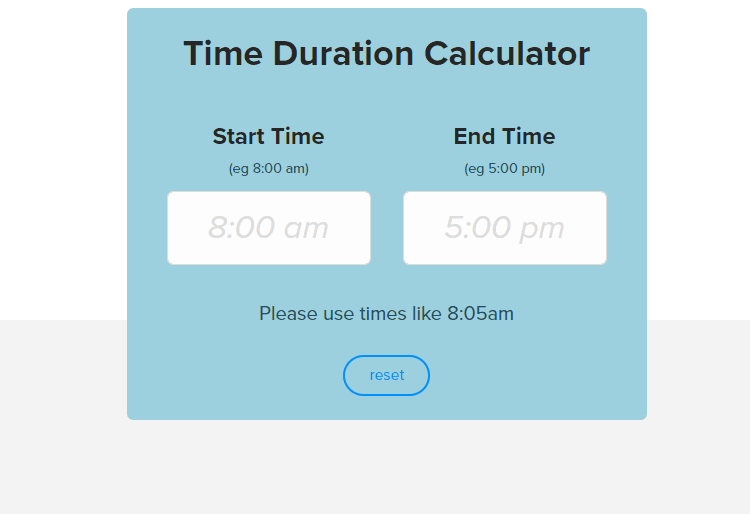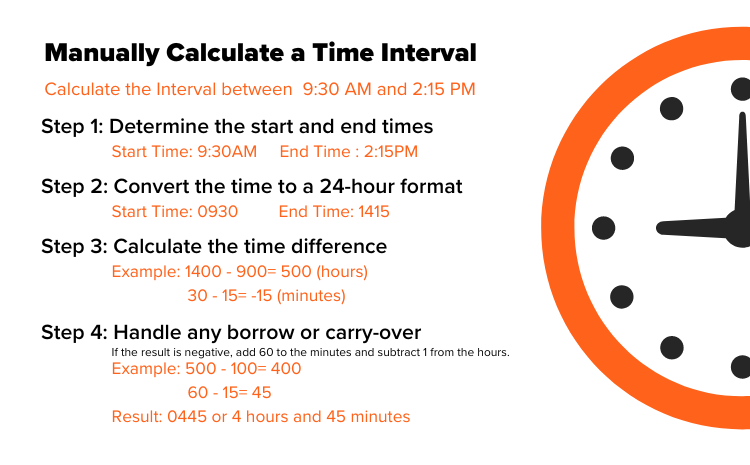# Time Duration Calculator

Calculate the duration between two times instantly with our easy-to-use online calculator.

(eg 8:00 am)

(eg 5:00 pm)

# 0 hours

or
0 hours

## Convert Time to a Decimal Format Instantly

If you regularly use a time duration calculator as part of a manual payroll process, it may be time to upgrade your tech.

OnTheClock’s cloud-based time clock keeps track of hours worked, automatically deducting breaks from the duration of a shift. No need for manual conversions — we can display time in military or standard format, as well as decimals or hours and minutes. Plus, our software seamlessly integrates with payroll providers, allowing you to process payroll in just a few clicks

Our time clock is simple to use and highly accurate, and can be accessed via a browser or mobile app. We’re also one of the most affordable time-tracking solutions. Accounts with 2 or fewer employees are free to use forever; subscription plans start at \$3.50 per user per month and are designed to affordably scale for growing businesses.

The best part? Your first 30 days of OnTheClock are free. Stop wasting time on manual payroll and start your free trial today.

## How to Calculate the Duration Between 2 Times

There are 2 basic methods to calculate the difference between 2 times. The easiest method is to use a time calculator to automatically determine the time duration. If you don’t have access to a time duration calculator (or just want to do the math manually), you’ll find instructions on how to manually calculate a time interval below.

## How to Use this Calculator

To use our time duration calculator, simply enter a start and end time to generate the result. The calculator will automatically generate the time duration in decimal hours and in hours and minutes. For example, let’s say your start time is 8:05 AM, and your end time is 12:15 PM. Your duration will be 4 hours and 40 minutes, or 4.67 hours.

We provide the time duration in decimals to make it easier to calculate payroll. Meanwhile, the hours and minutes format is generally used when communicating a time value verbally or in writing. If you already have a time duration and simply need to translate it to a decimal format, try our decimal hours calculator.### How to Manually Calculate a Time Interval

Manual calculations are time-consuming and prone to errors, so we recommend avoiding them when possible. Still, there may be occasions where you need to manually calculate a time duration interval. Here’s how;

Step 1: Determine the start and end times

Let’s say your start time is 9:30 AM and your end time is 2:15 PM.

Step 2: Convert the time to a 24-hour (a.k.a. military time) format

To do this, add 12 to the hours of any PM times, or use our military time converter. In this case, 9:30 AM would become 0930, and 2:15 PM would become 1415.

Step 3: Calculate the time difference

Subtract the start hours from the end hours to calculate the time difference (e.g., 1400 - 900 = 500). Then, do the same with minutes (e.g., 15 - 30 = -15).

Step 4: Handle any borrow or carry-overIf the minute value of the ending time is smaller than the minute value of the starting time, you'll need to "borrow" an hour from the hour value of the ending time. If the ending hour is a smaller value than the starting hour, the value of the difference will be a negative number. In this case, you should add 24 hours to the result.

So, in our example, since subtracting minutes from the ending time yielded a negative number (-15), we should add 60 to the minutes, then adjust the hours. So, instead of -15, we’ll have 45, and instead of 500, we’ll have 400.

First, add the minutes to the hours. From there, you can convert the format from military time to standard hours and minutes or to decimal format. In our example, the result is 445, which is 4 hours and 45 minutes, or 4.75 hours.

A

To calculate a time duration that contains 1 or more breaks, you’ll need to deduct the length of the breaks from your time interval. So, first, you should follow the steps above to calculate the entire length of a given duration. Then, you can subtract the length of the break from the total.

A

To calculate the duration of time in minutes, you need to consider the number of hours and minutes involved. To convert hours to minutes, multiply the number of hours by 60. Next, if you have any additional minutes beyond the whole hours, simply add them to the total.

So, for example, let’s say you’d like to convert 2 hours and 30 minutes into minutes. First, you would multiply 2 by 60, resulting in 120 minutes. Then, you’d add the remaining 30 minutes. The result is 150 minutes because 120 + 30 = 150.

A
• There are 168 hours in a week.
• There are 720 hours in a month with 30 days.
• There are 744 hours in a month with 31 days.
• There are 8,760 hours in a standard year.

The month of February has 672 hours in a standard year. During a leap year, February is 696 hours long. A leap year has 8,784 hours.

### Decimal hours calculator

Convert minutes to decimal hours and vice versa.